# How to use the COUNTIF function in Excel

The COUNTIF function of excel just counts the number of cells with a specific condition in a given range.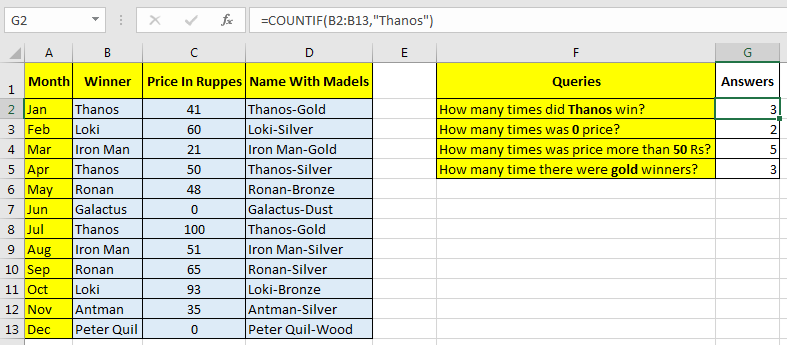Syntax Of COUNTIF Statement

=COUNTIF(range, condition)

Range: it is simply the range in which you want to count values.

Condition: This is where we tell Excel what to count. It can be a specific Text (should be in “”), a number, a logical operator(=,><,>=,<=,<>) and wild card operators (*,?).

Let's understand COUNTIF Statement with some easy examples

### COUNTIF to Count Text

Note: COUNTIF is not case sensitive. A and a, both are treated equally.
To count a specific text in range in excel, always use double quotes (“”). I have this data in a spread sheet.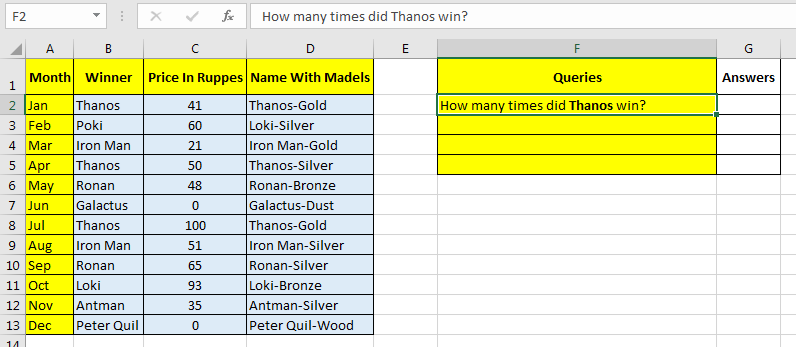In range A1:D13 i have my data. Now one query has arrived. How many times did Thanos win? In column B I have names of winners.
So in cell G2 I write this excel COUNTIF formula:

=COUNTIF(B2:B13,"Thanos")

The formula will return a number of times Thanos appears in the range of B2:B13.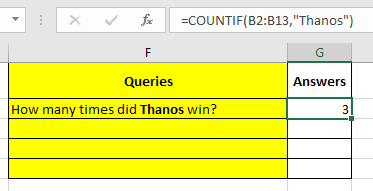### COUNTIF to Count Numeric Values

You don’t need to use double quotes (“”) to count numeric values. Let’s see an example first.
Now, after the first query, another query appears. How many times have there been 0 prices?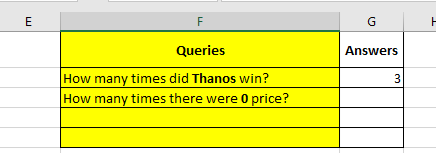To answer this, I wrote this COUNTIF formula in cell G3.

=COUNTIF(C2:C13,0)

And it returns 2. Because the price is in Range C2:C13, I used it in range.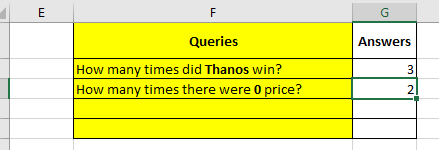### COUNTIF to Count Numbers with Conditions

After answering the last query, I got another query, i.e. How many times was the price more than 50 Rs?
So now we need to count all values that are greater than 50.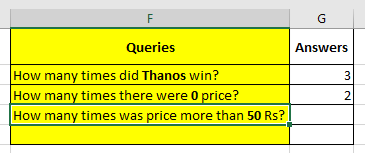While using numeric operators (=,>,<,>=,<=,<>) you need to use double quotes.
So now in cell G3, I wrote this COUNTIF formula:

=COUNTIF(C2:C13,">50")

It returns 5. Notice the double quote. Now, if your condition value was in some other cell, say in E4 than you would write:

=COUNTIF(C2:C13,">”&E4)

Either way, the result will be the same.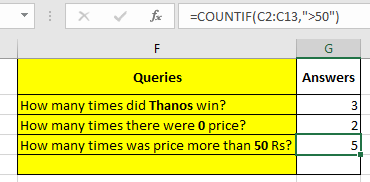## COUNTIF with Wild Card Operators (*,?)

### USE of * Operator In Excel COUNTIF FUNCTION

We have another query here. How many times have there been gold winners? Now it is mentioned in D. But it contains the names of the winners too.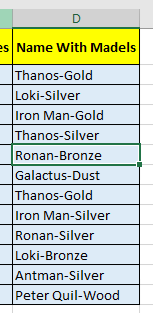If you write COUNTIF(D2:D13,”Gold”). It will return 0. Because no cell contains Gold according to excel. But it does. To tell Excel to count any value that ends with Gold, we would write this COUNTIF formula in cell G5:

=COUNTIF(D2:D13,"*Gold")

I will return the correct value which is 3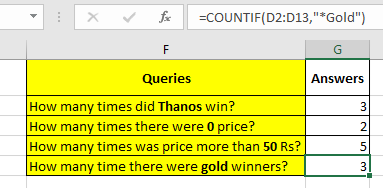Now the COUNTIF if function counts every value that ends with gold. The * operator just accepts anything written before Gold. If you write *Gold*, then COUNTIF will count anything that contains Gold in it.

### USE of ? Operator COUNTIF FUNCTION

The ? operator is used when you know how many letters there are, but you don’t know exactly what they are. For example, if I want to count all the Delhi pin numbers from a range, then I know they start at 1100 and are in total 6 digits. So my search criteria will be “1100??”.
Try this one by yourself and let me know what you found. Use the comments section below to ask questions and give suggestions. They are highly appreciated.

Related Articles:

How to Count Unique Values In Excel

How to use COUNTIFS with Dynamic Criteria Range in Excel

How to use COUNTIFS Two Criteria Match in Excel

COUNTIFS With OR For Multiple Criteria in Excel

How to use the COUNTIFS Function in Excel

How to Use Countif in VBA in Microsoft Excel

Popular Articles:

50 Excel Shortcuts to Increase Your Productivity

How to use the VLOOKUP Function in Excel

How to use the SUMIF Function in Excel

1.Hello. Can you please help me figure out how to count how many times a value occurs between two date columns?

I'm trying to count how many, let say "installs," occur per week with the start at end date columns.
There is more than one row with start dates and end dates that overlap and I'm trying to break it down by how many are overlapping in each week.
Column B & C are the start and end of the install. E and F are just part of my model.
For example, someone may have 44 installs per year, but how many are occurring each week at the same time.
I'm trying to show how many installs occur/overlap to the right of columns E and F via column G.